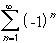#Interactive Real Analysis

Next | Previous | Glossary | Map

## 4.1. Series and Convergence

### Examples 4.1.5(a):

The seriesdoes not converge.
Consider the sequence of partial sums:
S n = -1 + 1 - 1 + 1 ... - 1 = -1
if n is odd, and
S n = -1 + 1 - 1 + 1 ... - 1 + 1 = 0
if n is even.

### You browser is not 'Java-enabled' ...

But then we have that
S n = -1 if n is odd and 0 if n is even
or, in other words, the sequence of partial sums is the same as the sequence
{ 1/2 ((-1)n-1)}
This sequence diverges, similar to the sequence {(-1)n} we discussed previously. Hence, our sequence of partial sums - while bounded - does not converge and therefore the series is divergent.
Next | Previous | Glossary | Map